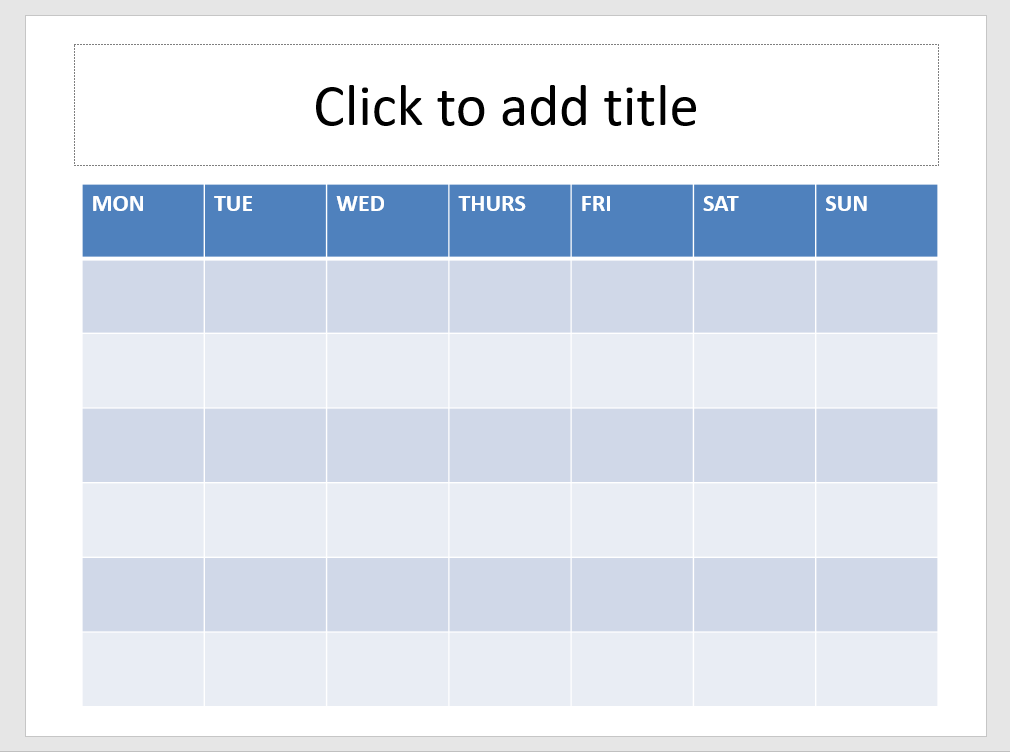PowerPoint tips, hints and tutorials that will change your presentations for ever!

INDEXHow to Make a Calendar for any Month or Year

Step One

Create a slide with Title and Text layout.

Add a Table 7 x 7

Fill in the first row as in the image.Now copy the code below.

Sub calendar_Update()

Dim lngY As Long
Dim lngM As Long
Dim firstDay As Long
Dim lngDayCNT As Long
Dim lastDay As Long
Dim lngDay As Long
Dim lngCount As Long
Dim X As Long
Dim L As Long
Dim osld As Slide
Dim otbl As Table
Dim LR As Long
Dim LC As Long
Dim rayDays(1 To 42) As String
Const StartDay As Long = vbMonday

On Error Resume Next
Set otbl = ActiveWindow.Selection.ShapeRange(1).Table
If otbl Is Nothing Then
MsgBox "Select a table", vbCritical
Exit Sub
End If

' Get year and month
lngY = InputBox("Enter Year")
If Not IsNumeric(lngY) Then Exit Sub
lngM = InputBox("Enter Month Number (e.g. 2 for February")
If Not IsNumeric(lngM) Then Exit Sub
If lngM < 1 Or lngM > 12 Then Exit Sub

' Find day of week for 1st of month
firstDay = Weekday(DateSerial(lngY, lngM, 1), StartDay)
' Find number of days in month
lngDayCNT = Day(DateSerial(lngY, lngM + 1, 1) - 1)
' find day of week for last day
lastDay = lngDayCNT + firstDay - 1
'add only used days to array
For L = firstDay To lastDay
lngDay = lngDay + 1
rayDays(L) = lngDay
Next L
' fill in Table omit header row

For LR = 2 To 7
For LC = 1 To 7
X = X + 1
otbl.Cell(LR, LC).Shape.TextFrame.TextRange = CStr(rayDays(X))
otbl.Cell(LR, LC).Shape.TextFrame.TextRange.Font.Size = 10
Next
Next
Set osld = ActiveWindow.Selection.SlideRange(1)
osld.Shapes.Title.TextFrame.TextRange = MonthName(lngM) & " " & lngY

End Sub

Step Two

Open the VB editor by pressing ALT f11

INSERT > Module and paste the code into the module

Step 3

Go back to the main window and run the code from View > Macros. Fill in the Year and Month and your are done

If you are looking for 2020 / 2021 versions of MSFT calendars try HERE

Back to the Index Page